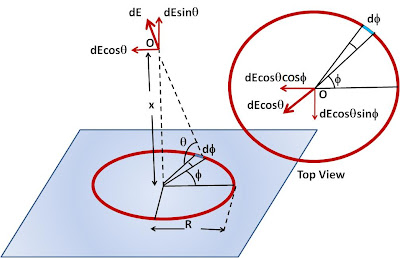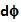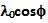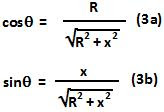## Sunday, January 31, 2010

### Irodov Problem 3.12Consider an infinitesimally small section of the ring that subtends an angleand is located at angle. The length of this infinitesimally small section of the ring is. Since the linear charge density of the ring depends onas, the total charge contained in this infinitesimally small section of the ring is given by,The electric field generated by this infinitesimally small section of the ring at point O, as depicted in the figure, is then given by,As seen from the Figure, using elementary trigonometry we See that,From (1), (2), (3a) and (3b) we obtain,The field at the center of the ring can be obtained by setting x=0 as,